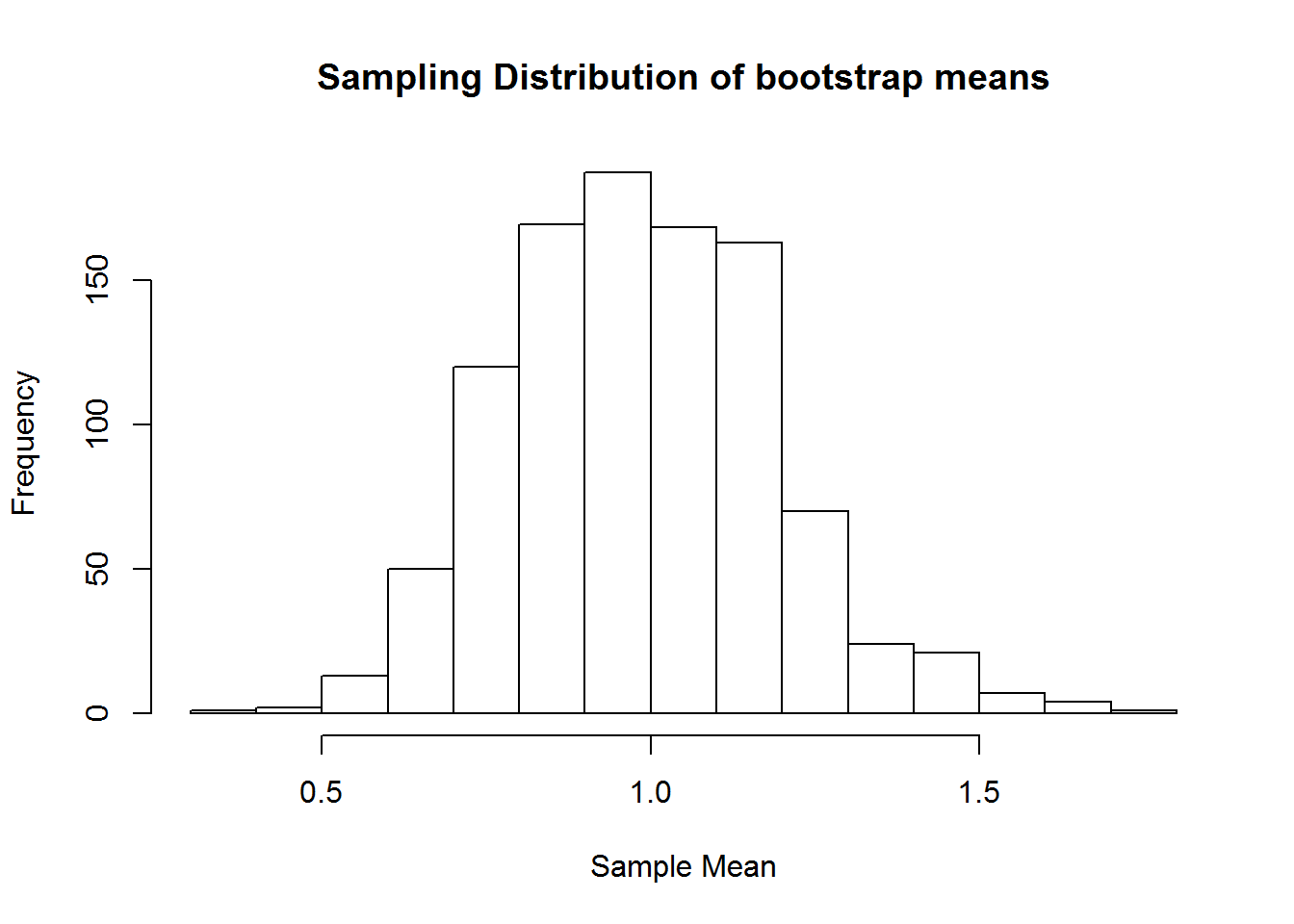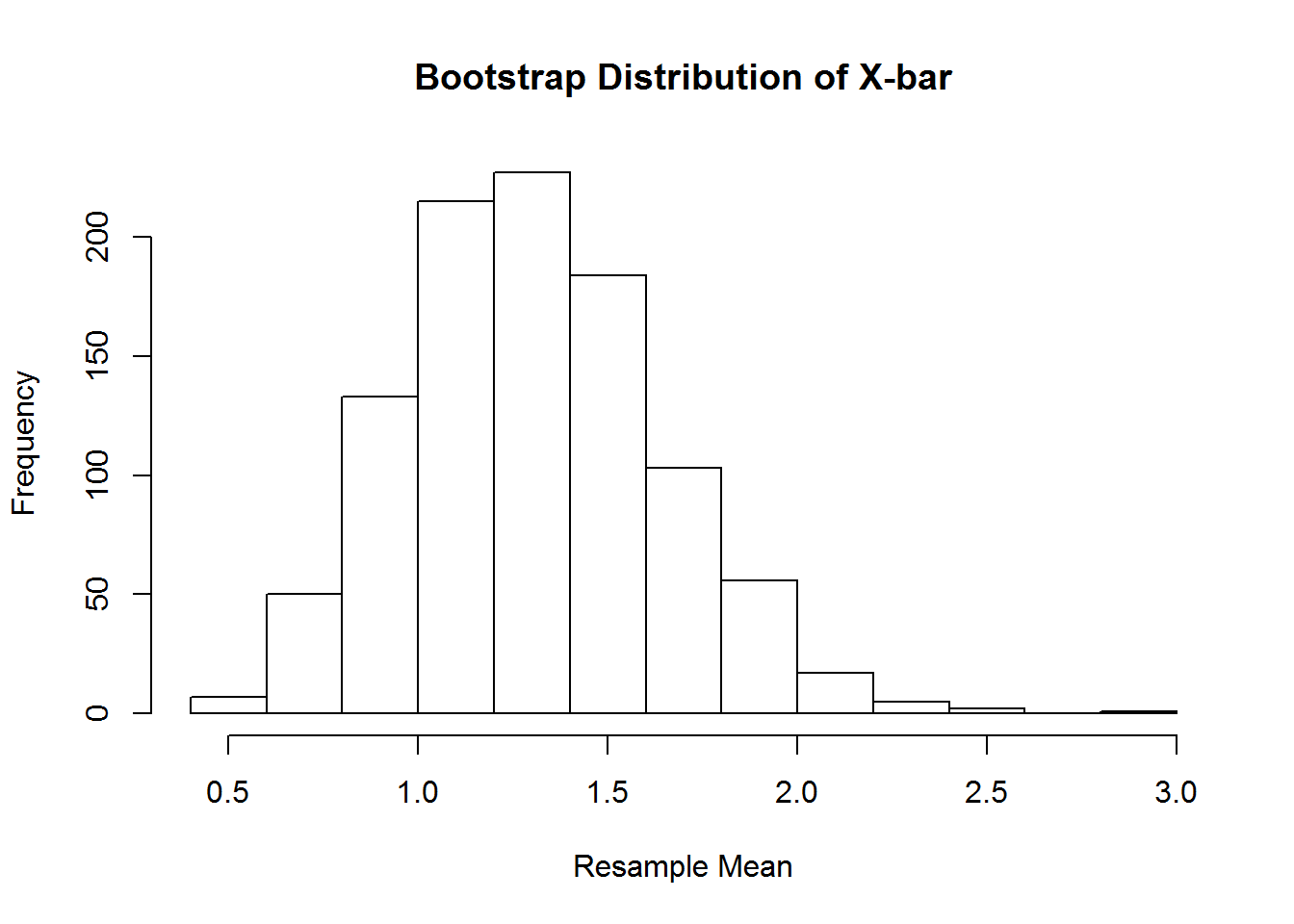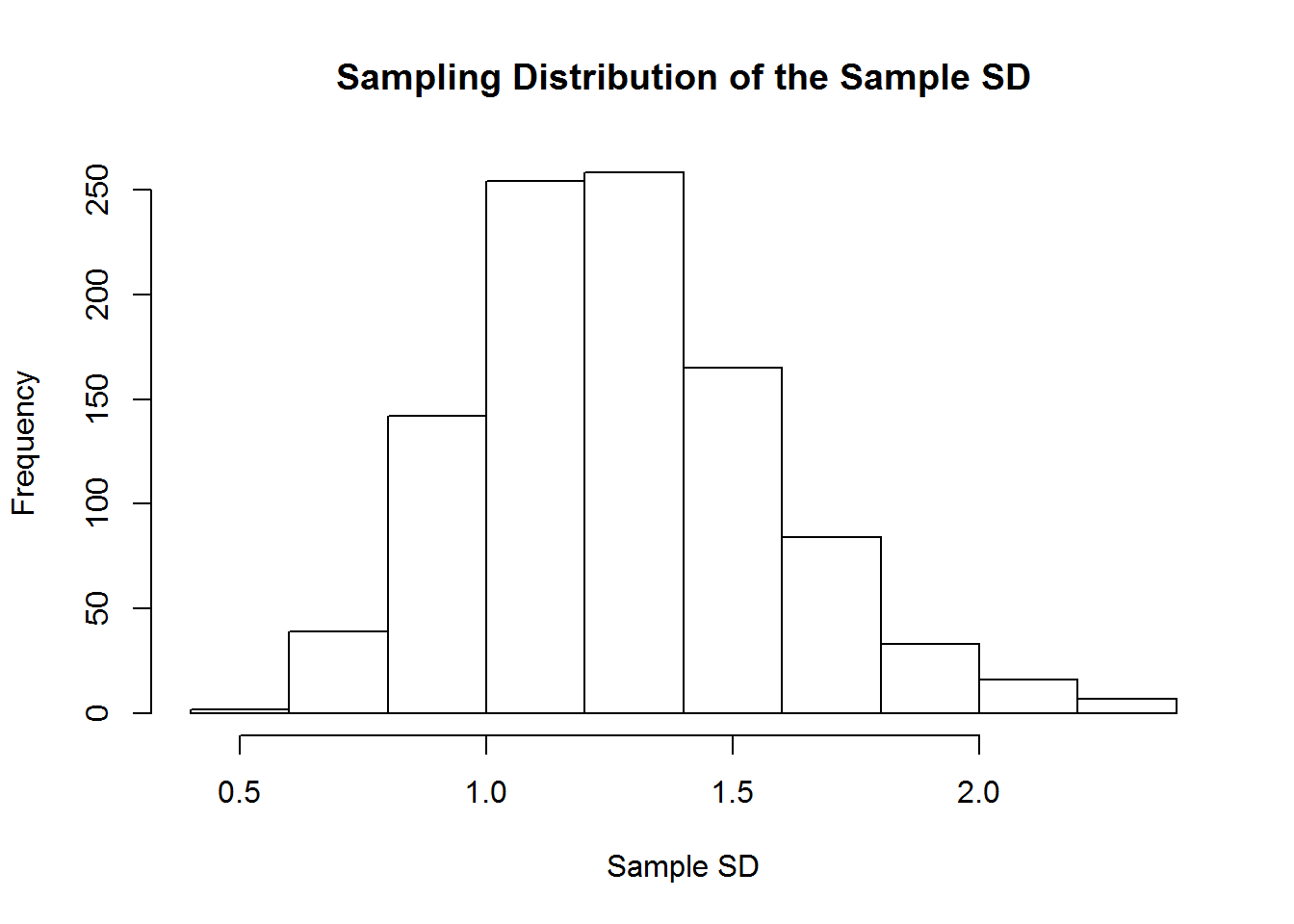C1_ChiSq <- read_csv("P:/SUNY-Brockport/SUNY Brockport_1/teaching/Staitstical Methods/Fall2019/Chapter1RLab/Bootstrap/Bootstrap/C1ChiSq.csv",col_names = FALSE)
## Parsed with column specification:
## cols(
##   X1 = col_double()
## )
View(C1_ChiSq)

# Construct and observe the histogram of the sampling distribution of X: based on mean of 1000 samples WOR of size n=40 from chisq data

reps <- 1000
means <- numeric(reps)
n<-40
y<-C1_ChiSq\$X1
for (i in 1:reps) {
means[i] <- mean(sample(y,n))
}
hist(means,main="Sampling Distribution of bootstrap means",xlab="Sample Mean")#Mean and standard deviation of the sampling distribution of X :
bootstrap.mean<-mean(means)
bootstrap.sd<-sd(means)

# Construct the bootstrap distribution of X based on 1000 resamples WR of one simple random sample of size n=40

samp.chisq <- sample(y,n)
reps <- 1000
means1 <- numeric(reps)
for (i in 1:reps) {
means1[i] <- mean(sample(samp.chisq,n,replace=TRUE))
}
hist(means1,main="Bootstrap Distribution of X-bar",xlab="Resample Mean")# 95% Bootstrap Percentile Confidence Interval for the population mean:

LCL.t<-round(mean(means)-qt(.975,n-1)*sd(means1),2)
UCL.t<-round(mean(means)+qt(.975,n-1)*sd(means1),2)
#note that we use the mean from original sample and bootstrap distribution to edtimate the spread

LCL.p<-round(quantile(means1,.025),2)
UCL.p<-round(quantile(means1,.975),2)
CI.Table<-cbind(LCL.t,UCL.t, LCL.p,UCL.p)
CI.Table
##      LCL.t UCL.t LCL.p UCL.p
## 2.5%   0.3  1.67  0.72     2

# Construct the sampling distribution and bootstrap distribution of the sample standard deviation:

reps <- 1000
sds <- numeric(reps)
for (i in 1:reps) {
sds[i] <- sd(sample(y,n))
}
hist(sds,main="Sampling Distribution of the Sample SD",xlab="Sample SD")